### AME 301: Differential Equations, Vibrations and Control Homework 10

 B. GoodwineJ. Lucey Fall, 2003 Issued: November 21, 2003 Due: November 26, 2003

Unless otherwise specified in this homework, you may assume that all initial conditions for a system are zero.

1. Consider the inverted pendulum illustrated in the following figure (you may consider the mass to be an idealized point mass).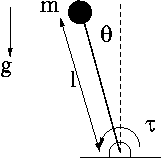1. (5 points) Determine the equation of motion for the system and the linearization of the equation of motion about the equilibrium position when the pendulum is vertical.
2. (5 points) Determine the transfer function from the input torque to the output angle, i.e., find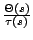.
3. (5 points) Determine the transfer function from the input torque to the output angular velocity, i.e., find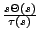.
4. Assume the torque is supplied by a d.c. motor, as illustrated in the following figure.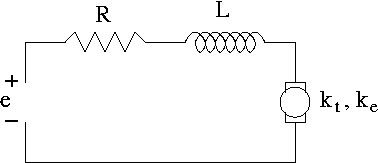1. (5 points) Determine the transfer function from the applied voltage to the output angle, i.e., find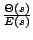.
2. (5 points) Determine the transfer function from the applied voltage to the output angular velocity, i.e., find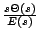.

2. Consider the mass-pulley system illustrated in the following figure (assume the system is planar, i.e., no gravity).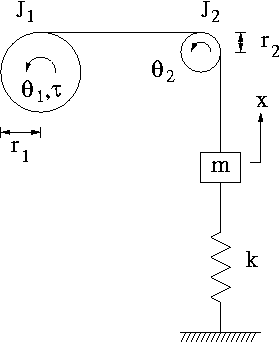1. (5 points) Determine the equation of motion for the system. Hint: in addition to using Newton's law on the mass and two pulleys, assume that the cord does not slip on the cable. That assumption will provide two equations: one relating the angle of pulley 1, the radius of pulley 1, the angle of pulley 2 and the radius of pulley 2 and another relating the angle and radius of pulley two and the displacement of the mass (these are known as kinematic constraints).
2. (5 points) Determine the transfer function from the torque to the position of the mass, i.e., determine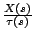.
3. (5 points) Determine the transfer function from the torque to the velocity of the mass, i.e., determine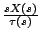.
4. Assume the torque is supplied by a d.c. motor, as illustrated in the following figure.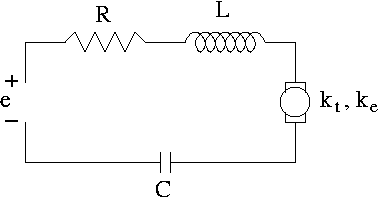1. (5 points) Determine the transfer function from the applied voltage to the position of the mass, i.e., find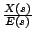. Hint: you may want to (perhaps even need to) add a variable for the voltage drop across the capacitor and then add an equation relating the current through the capacitor to the voltage across it.
2. (5 points) Determine the transfer function from the applied voltage to the velocity of the mass, i.e., find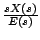.
3. (5 points -- extra credit) Determine the transfer function from the current in the circuit to the position of the mass, i.e., find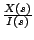.
4. (5 points-- extra credit) Determine the transfer function from the current in the circuit to the velocity of the mass, i.e., find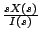.
3. (10 points) Find the transfer function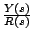for the following block diagram.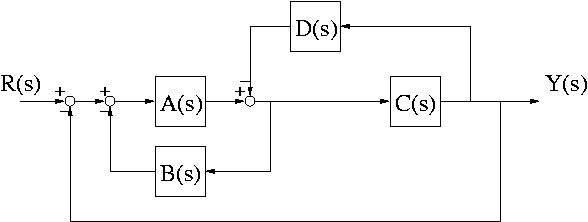4. (10 points) Find the transfer functionfor the following block diagram.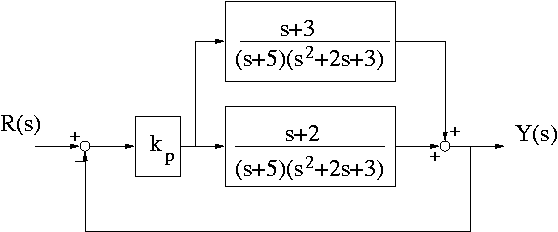It thus relativizes discourse not just to form--that familiar perversion of the modernist; nor to authorial intention--that conceit of the romantics; nor to a foundational world beyond discourse--that desperate grasping for a separate reality of the mystic and scientist alike; nor even to history and ideology--those refuges of the hermeneuticist; nor even less to language--that hypostasized abstraction of the linguist; nor, ultimately, even to discourse--that Nietzschean playground of world-lost signifiers of the structuralist and grammatologist, but to all or none of these, for it is anarchic, though not for the sake of anarchy but because it refuses to become a fetishized object among objects--to be dismantled, compared, classified, and neutered in that parody of scientific scrutiny known as criticism.

---Stephen Tyler, Writing Culture, James Clifford and George E. Marcus, editors.

Last updated: November 21, 2003.
B. Goodwine (goodwine@controls.ame.nd.edu)
J. Lucey (jlucey@nd.edu)